# Test: Electrostatic Potential & Potential due to a Point Charge (NCERT)

## 10 Questions MCQ Test NCERTs for NEET: Textbooks, Tests & Solutions | Test: Electrostatic Potential & Potential due to a Point Charge (NCERT)

Description
Attempt Test: Electrostatic Potential & Potential due to a Point Charge (NCERT) | 10 questions in 10 minutes | Mock test for NEET preparation | Free important questions MCQ to study NCERTs for NEET: Textbooks, Tests & Solutions for NEET Exam | Download free PDF with solutions
QUESTION: 1

### Which of the following statement is not true?

Solution:

Work done by the electrostatic force is independent of the path followed by it, and it depends only on the initial and final positions. For example, work done in moving a unit positive charge in a closed loop of an electric field is zero.

QUESTION: 2

### 1 volt is equivalent to

Solution:

Volt is the electrical unit of potential difference or voltage, and is expressed as V. One Volt is described as one joule of energy consumption per one coulomb electric charge.

QUESTION: 3

### The work done in bringing a unit positive charge from infinite distance to a point at distance x from a positive charge Q is W. Then the potential ϕ at that point is

Solution:

Electric potential at a point in an electric field is defined as the work done in bringing a unit positive charge from infinity to that point.
Or,  V = W/q ​
Since charge is of magnitude unity, Hence electric potential at that point will be equal to the workdone ∴ ϕ = W

QUESTION: 4

The potential at a point due to a charge of 5×10−7C located 10cm10cm away is

Solution:

Here, q = 5 × 10(−7) C, r = 10 cm = 0.1 m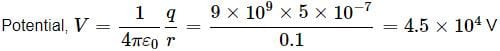QUESTION: 5

In the question number 4, work done in bringing a charge of 4 x 10-9 C from infinity to that point is

Solution:

Work done, W = q(Vf − Vi) = 4 × 10−9 × 4.5 × 104 = 1.8 × 10−4 J

QUESTION: 6

The electric field intensity at a point P due to point charge q kept at point Q is 24 N C−1 and the electric potential at point P due to same charge is 12 J C−1. The order of magnitude of charge q is

Solution:

Electric field of a point charge.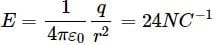Electric potential of a point charge,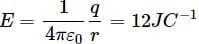The distance PQ is r = V/E = 12/24 = 0.5 m
∴ Magnitude of charge
q' = 4πε0Vr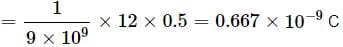= 10−9 C

QUESTION: 7

The electric potential at a point in free space due to charge Q coulomb is Q x 1011 volts. The electric field at that point is

Solution:

The electric potential due to charge Q is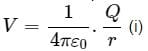where r is the distance of observation point from the charge. At the same point, electric field is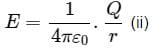Combining Eqs. (i) and (ii), we have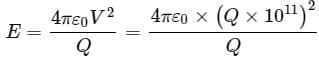= 4πε0Q × 1022 V/m-1

QUESTION: 8

Two points A and B are located in diametrically opposite directions of a point charge of +2μC at distances 2m and 1m respectively from it. The potential difference between A and B is

Solution:

Here, q = 2 μC = 2 × 10−6 C,
rA = 2m, rB = 1m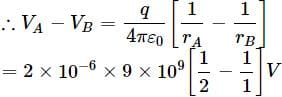= −9 × 103  V

QUESTION: 9

A charge +q is placed at the origin O of x-y axes as shown in the figure. The work done in taking a charge Q from A to B along the straight line AB is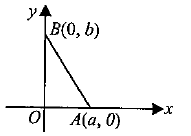Solution:

Potential at point A is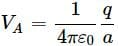Potential at point B is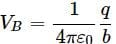Work done in taking a charge Q from A to B is,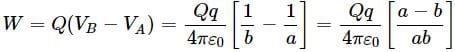QUESTION: 10

As per this diagram a point charge +q is placed at the origin O. Work done in taking another point charge −Q from the point A [coordinates (0, a)] to another point B [coordinates (a, 0)] along the straight path AB is

Solution:

Work done is equal to zero because the potential of A and B are the same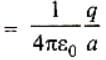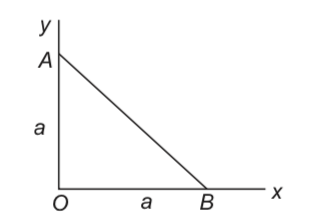No work is done if a particle does not change its potential energy
i. e. initial potential energy = final potential energy.Use Code STAYHOME200 and get INR 200 additional OFF Use Coupon Code